# MATLAB Review

## 26/09/2018

This is a MATLAB tutorial guide for the course Mathematical Modeling (MATH3290) in Chinese University of Hong Kong (CUHK). All the codes can be executed on MATLAB 2016a (or version above). It requires MATLAB 2016a (or version above) to open this live script.
There are many applications for MATLAB. In this guide, we focus on the following topics:
• Basic operations in MATLAB;
• Common built-in functions in MATLAB;
• Flows of control, including if-else, for/while-loop;
• Basic plotting: how to visualize your results;
• Debugging and getting help.
Finally, we provide some (programming) practice problems related to our course.

# Basic operations in MATLAB

## Addition (or subtraction)

% Between scalars
3 + 5
3.14 + 1.57
2/7 + 0.16
format short
2/7 + 0.16
% Between scalar and vector (or matrix)
1 + [1;1;1;1]
I = 1 + [1,0;0,1]
disp(I)
% Between vectors / matrice
[1;2;3;4] - [5;6;7;8]
new_vector = [1;2;3;4]

## Remark

1. Each row of matrix end with the sign ";".
2. For subtraction, just replace "+" by "-" with exactly the same syntax.
3. It is useful to grasp part of the matrix using the sign ":" and the keyword "end".
A = magic(5)
A1 = A(2:3, 4:5)
disp(A1)
A2 = A(1:3,:)
disp(A2)
A3 = A(:,1:3)
disp(A3)
A4 = A(:,2:end)
disp(A4)

## Multiplication

% Between scalars
2 * 4
3.14 * 1.57
format short
3.14 * 1.57
format long
ans
2.7 * 3/2
format short
ans
% Between scalar and vector (or matrix)
3.5 * [1;1;1;1]
pi * [1,2;3,4]
format long
pi
% use frpintf to print out more digits
% Between matrice (MATRIX-MULTIPLICATION)
[1 2 3] * [4;5;6]
[1 2 3; 4 5 6] * [7 8; 9 10; 11 12]
% Between matrice (ENTRY-WISE)
A = [1 2 3; 4 5 6]
B = [7 8 9; 10 11 12]
A .* B
a = A(1,:)'
b = A(2,:)'
sum(a.*b)
a'*b

## Division (Inversion)

r1 = 2 / 7
r2 = 2 \ 7
A = [1 2; 4 5]
B = [7; 10]
x = A\B
% A x = B;
format rat
invA1 = A^(-1)
invA2 = inv(A)
disp(invA1)
disp(invA2)
Remark: We prefer to use x = A\B rather than x = inv(A)*B.

## Power

p1 = 2^5
p2 = power(2,5)
A = [1 2; 4 5]
B = [3 4; 5 6]
result = A .^ B
disp(A)
disp(B)
disp(result)
a = 27^(1/3)
b = nthroot(27,3)
c = 16^(1/2)
d = sqrt(16)

## Summary

• Basic operations including: addition, subtraction, multiplication, division and power.
• Display format in MATLAB: short, long, rat, bank, etc.
• For some operations (e.g., multiplication or power) there are entry-wise versions .* or .^.
• If you are doing the entry-wising operation, the two operants have to be same size.
• One may solve Ax = b by using the operation "\" in MATLAB: x = A\b.

# Common built-in functions in MATLAB

There are many useful built-in functions in MATLAB and we just name very few of them here, which are frequently used in this course.
• zeros, ones, repmat, kron, linspace, diag, eye;
• mean, norm, sum, abs, max, min, det, rank;
• rand, randn, mod, floor, round, ceil, eig;
• who, whos, clear, clc;
• plot, scatter;
• disp, save, load;
zero_mat = zeros(3,4);
disp(zero_mat)
ones_mat = ones(3,4)
disp(ones_mat)
A = ones(2,1);
A_rep = repmat(A,1,2)
disp(A)
disp(A_rep)
random_field = rand(3,3);
disp(random_field)
Users may want to define a function by themselves. There are two common ways to do so.
% Function handle
% function_name = @ (list of arguments) mathematical expressions
clear a b c;
quad_root = @(a,b,c) [(-b+sqrt(b^2-4*a*c))/(2*a) (-b-sqrt(b^2-4*a*c))/(2*a)];
r = quad_root(1,2,1)
disp(r)
p = quad_root(1,-3,2)
disp(p)
% Define a function in a separate m-file.
[x1,x2] = quad_solver(1,2,1)
% function [x1,x2] = quad_solver(a,b,c)
% x1 = (-b+sqrt(b^2-4*a*c))/(2*a);
% x2 = (-b-sqrt(b^2-4*a*c))/(2*a);
% end

# Flows of control

## If-else statement.

The logical expression can be some variables, inequality or equality. We list some symbols used in logical expressions.
• <, less than;
• >, greater than;
• <=, less than or equal to;
• >=, greater than or equal to;
• ==, equal to;
• ~=, not equal to.
% if-else
% Syntax
% if (logical expression)
% program statements ...
% end
% if (logical expression)
% program statements ...
% else
% another program statements ...
% end
a = 0; b = 0; c = 1;
if (b^2 - 4*a*c) >= 0
disp('This quad-eqn has two real roots.');
else
disp('This quad-eqn has two imaginary roots.');
end
a = 1; b = 0; c = 1;
if (b^2 - 4*a*c) >= 0
disp('This quad-eqn has two real roots.');
else
disp('This quad-eqn has two imaginary roots.');
end

## For-loop statement

We use for-loop in MATLAB in order to execute some commands specific number of times. Usually, some_vector may be 1:1:n, where n is a large integer.
% For-loop
% for idx = some_vector
% program statements ...
% end
% Example: Forming Hilbert matrix without built-in function.
% Plotting the condition number of Hilbert matrix against its size.
% cond(H) = || H ||_2 * ||H^(-1)||_2
% || H ||_2 = max_{x neq 0 in R^n} ||Hx||_2 / ||x||_2.
cond_H = [];
for sz = 1:1:10
H = zeros(sz,sz);
for m = 1:sz
for n = m:sz
H(m,n) = 1/(m+n-1);
H(n,m) = H(m,n);
end
end
cond_H = [cond_H cond(H)];
end
scatter(1:10,log10(cond_H))
title('Condition number of Hilbert matrix','FontSize',16)
xlabel('Size of matrix','FontSize',14)
ylabel('Log10 of Condition number','FontSize',14);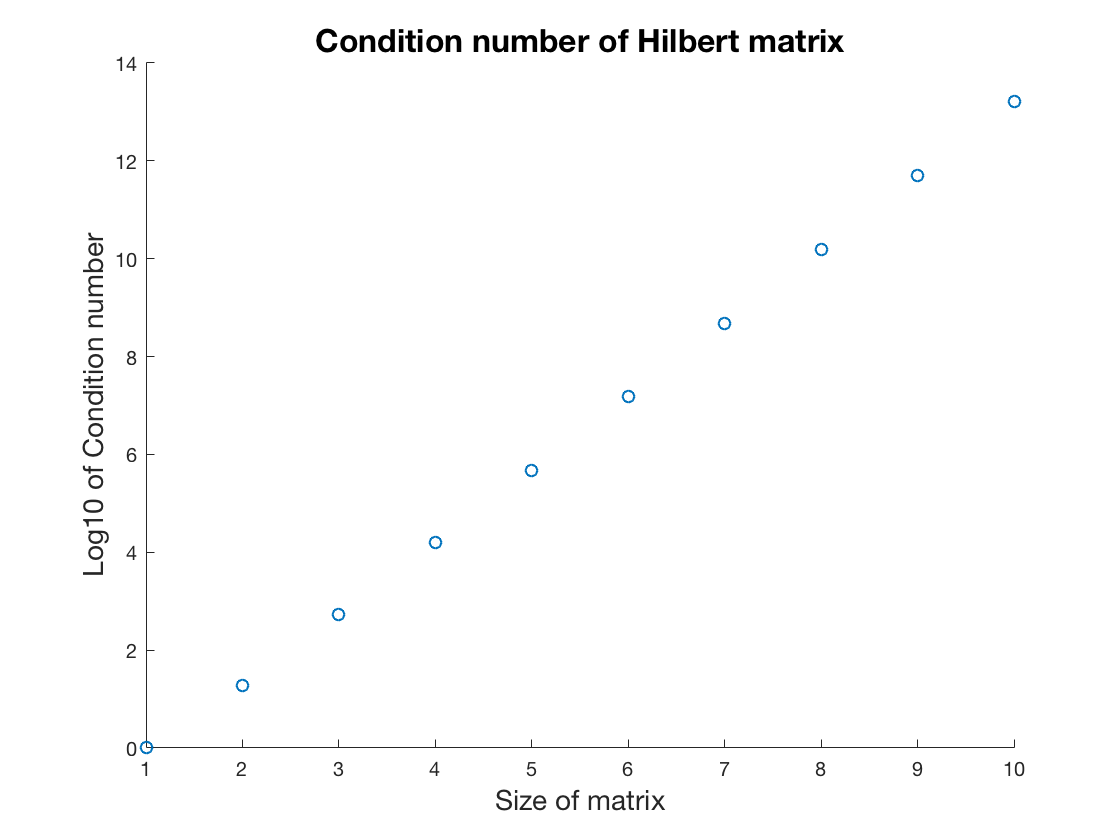## While-loop statement

We use while-loop to execute (repeatedly) when the condition is true.
• If the experssion is true, the program statements will be excuted;
• After finishing all statements, the expression on top will be determined again;
• The program will keep being run if expression is true, otherwise terminate.
% While-loop
% while expression
% program statements ...
% ... ...
% end
% For example, calculating sin(x) by power series up to certain number of power.
x = 2; s = 1; max_power = 10;
% sin(x) \approx x/1! - x^3/3! + x^5/5! - x^7/7! + x^9/9!
format short;
res = 0;
while s <= max_power
res = res + (-1)^((s-1)/2)*(x.^s)/(factorial(s));
s = s+2;
end
s
res
error = abs(res - sin(x))

# Basic plotting

Visualizing your results is important. A graph is better than a thousand of words. One may sketch some figures by MATLAB in assignments if necessary.
First, we look at a typical example of mathematical modeling. Let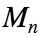be the population of mouse after n years and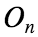the population fo owl after n years. The ecologist has suggested the following modelWe want to know if two species can coexist in the habitat and if the outcome is sensitive to the starting population. Given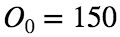and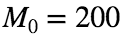. How does the population of two species change after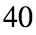years?
M = 200; O = 150;
n = 40;
record = zeros(2,n+1);
record(:,1) = [M;O];
for i=2:n+1
M_new = 1.2*M - 0.001*O*M;
O_new = 0.7*O + 0.002*O*M;
record(:,i) = [M_new;O_new];
M = M_new;
O = O_new;
end
How can one show the results? Use the built-in function plot to sketch the figures. Further, one can set different kind of properties of the figure such as title, label and legend. One may also save the figure as a fig.file using the built-in function saveas.
fh = figure;
h = plot(1:(n+1),record(1,:),1:(n+1),record(2,:));
set(h(1),'LineWidth',2); set(h(2),'LineWidth',2);
set(h(1),'Marker','*'); set(h(2),'Marker','s');
set(h(1),'Color','b'); set(h(2),'Color','r');
h_l = legend('Mice', 'Owl');
set(h_l, 'Location', 'NorthWest');
title('Owls vs Mice','FontSize',16);
xlabel('Number of years','FontSize',14);
ylabel('Population','FontSize',14);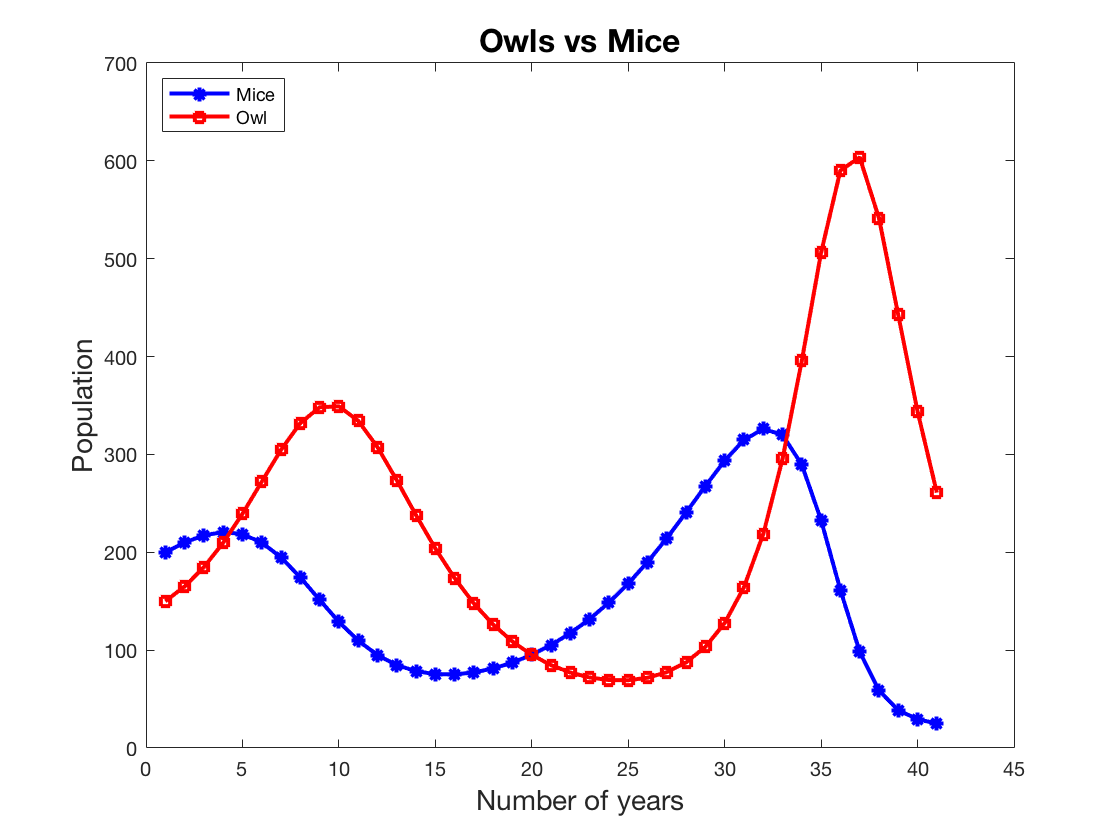% saveas(fh,'assignment_plot','fig');

# Application: Cubic splline

In this section, we investigate in MATLAB the calculation of a cubic spline, which is a commonly used mathematical tool in our course.
Consider the following data setand find the natural cubic spline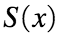that satisfies the data above. Assume thatNext, list all the conditionssatisfiesSolve the above linear system to obtain the coefficientsWe formulate the above linear system in MATLAB.
x = [3 4 5]; y = [12 17 38];
sz = size(x,2); % sz = 3;
b_c = zeros(4*(sz-1),1);
b_c([1;2;4]) = y; b_c(3) = y(2); % b_c = [12; 17; 17; 38; 0;0;0;0]
A_c = zeros(4*(sz-1),4*(sz-1));
A_c(1,1:4) = x(1).^(3:-1:0); % 3:-1:0 = [3 2 1 0];
A_c(2,1:4) = x(2).^(3:-1:0);
A_c(3,5:end) = x(2).^(3:-1:0);
A_c(4,5:end) = x(3).^(3:-1:0);
A_c(5,1:3) = (3:-1:1).*(x(2).^(2:-1:0));
A_c(5,5:end-1) = -A_c(5,1:3);
A_c(6,1:2) = ([6:-4:2]).*(x(2).^(1:-1:0));
A_c(6,5:6) = -A_c(6,1:2);
A_c(7,1:2) = (6:-4:2).*(x(1).^(1:-1:0));
A_c(8,5:6) = (6:-4:2).*(x(3).^(1:-1:0));
x_c = A_c \ b_c; % A_c * x_c = b_c;
Then, we obtain the coefficients x_c: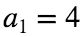,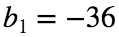,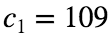,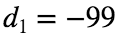,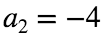,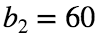,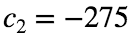and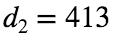.
One can sketch the graph of the cubic spline. Note that it is differentiable, especially at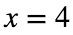.
xd1 = 3:0.01:4; xd2 = 4:0.01:5;
% xd1 = [3 3.01 3.02 3.03 ... 4]
% xd2 = [4 4.01 4.02 4.03 ... 5]
yd1 = x_c(1)*(xd1.^3) + x_c(2)*(xd1.^2) + x_c(3)*(xd1) + x_c(4)*(xd1.^0);
yd2 = x_c(5)*(xd2.^3) + x_c(6)*(xd2.^2) + x_c(7)*(xd2) + x_c(8)*(xd2.^0);
plot(xd1,yd1,'b-','LineWidth',1.0);
hold on;
plot(xd2,yd2,'m-','LineWidth',1.0);
scatter(x,y,'ro','filled');
legend('S_1(x)','S_2(x)','Location','northwest');
title('Figure of S(x)','FontSize',16);
xlabel('x','FontSize',14);
ylabel('y','FontSize',14);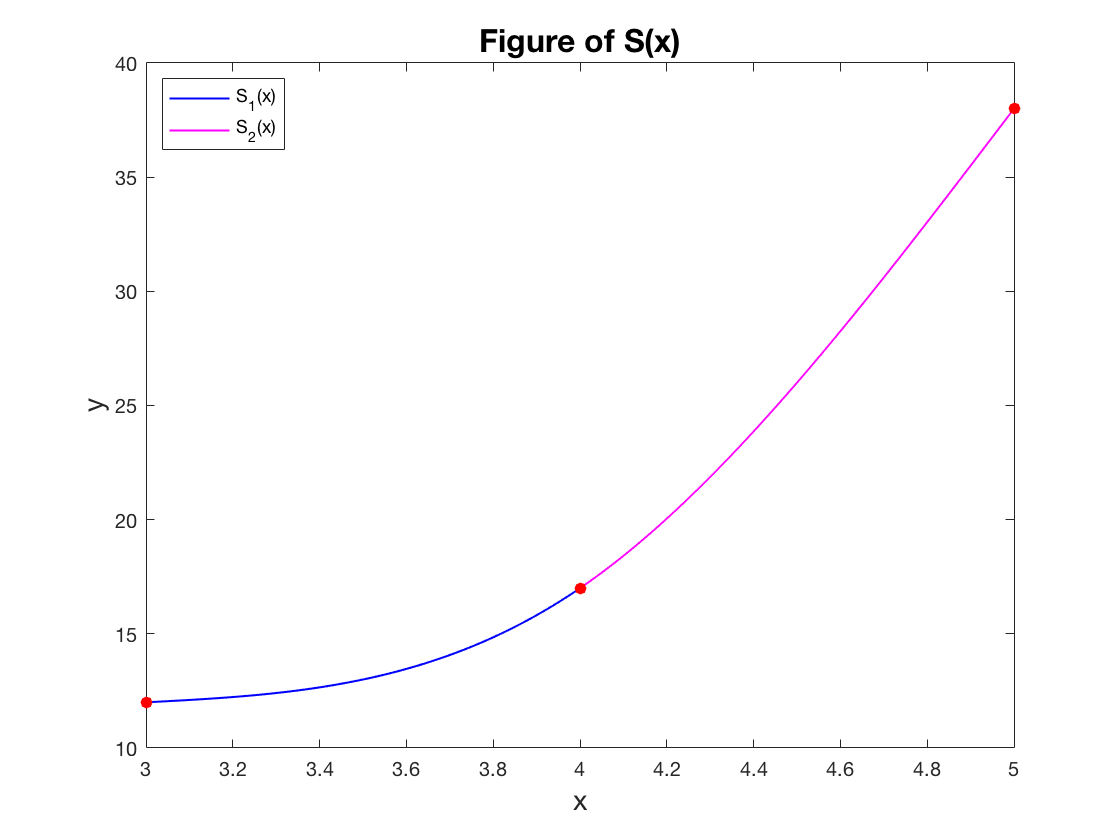# Debugging and getting help

## How to debug?

One may usually have different kinds of bugs or error in the program when coding. The bugs may come from the error of syntax, which is relatively easy to find out. Another type of bugs may be logically wrong and it has correct syntax, leading to unexpected results, and this is usually harder to figure out.
Let us look at a simple example: quadsolver() and we test two cases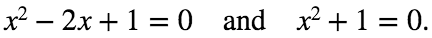[x1,x2] = quadsolver1(1,-2,1);
This is good to know. Next, we try another case.
[x1,x2] = quadsolver1(1,0,1)
Why would it lead to the unexpected result? It should be the case that
This quad-eqn has two imaginary roots.
Go down to the script of the function of quadsolver and see what happens. One may set breakpoint in the program (if under the usual script environment) so that one may see how the program runs step by step.
One may define another function called check_quadsolver and verify the correctness of our code.
d = check_quadsolver(1,0,1)
After that, one may fix the code suitably and now check the final result using the function quadsolver2.
[x1,x2] = quadsolver2(1,0,1)
Problem fixed.
Note that the logical sign ">=" or "<=" only makes use of the real part of a complex number and one should avoid to compare the amount between a complex number and another quantity, no matter this quantity is complex or not.
There is no the concept of "order" in complex field.
You can do it in real field. (5>1, -5 < -1.35 ...)

## Get help from MATLAB

The most efficient way to find a solution for a specific MATLAB issue is to use the keyword "help" or "doc" in MATLAB. For example, to explore the properties of the function "plot", one can type the following command to get some help.
help plot
Another way is to search the answer from internet, especially from Google or Stackexchange. Try to use accurate keywords to represent your problem. Note that, before asking any question, one should make the question as clear as possible and let people find it easier to provide the answer.

# Practice problems

## Problem 1

Consider the ordinary differential equation with initial conditionHere,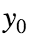is given. One may use Euler's method to numerical solve this ODE and this scheme is as follows: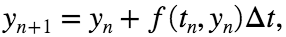where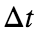is a given step size of time,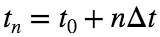and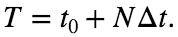In this problem, assume that the function and initial conditions areTake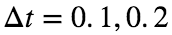and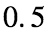and write a program to solve the initial value problem. Record all the values of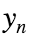and plotagainst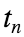. Also, compare the numerical solution to the exact solution of the initial value problem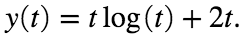Hint: To compare the accuracy, one may define a functional handle
g(t) = t*log(t) + 2*t and the vector variable error = abs(g(tn) - yn), where yn is the numerical approximation.

## Problem 2

Consider the unconstrained minimization problem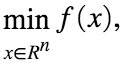where f is a differentiable (convex) function. Use classical gradient method to solve the problem. Choose an initial guess $x^0 = 0$ and repeat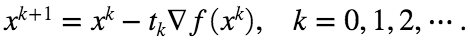Note that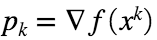is a descent direction and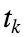is a step size. To determine, using the line search strategy as follows: initializeat some fixed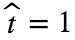, repeat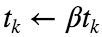until the following condition holds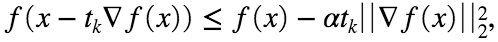where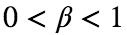and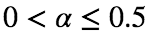and user-defined. Your task is to implement the gradient method with the following objective functionPlot a graph with the function value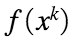against the iteration index k.

# Appendix: definition of the funtions in this script.

function [x1,x2] = quadsolver1(a,b,c)
d = sqrt(b^2-4*a*c);
if (d >= 0)
disp('This quad-eqn has two real roots.');
else
disp('This quad-eqn has two imaginary roots.');
end
x1 = (-b +d )/ (2*a);
x2 = (-b -d )/ (2*a);
end
function d = check_quadsolver(a,b,c)
d = sqrt(b^2-4*a*c);
disp('The delta is ');
disp(d);
s2 = sprintf('The logical expression is %d.', (d>=0));
disp(s2);
end
function [x1,x2] = quadsolver2(a,b,c)
d = b^2 - 4*a*c;
if (d >= 0)
disp('This quad-eqn has two real roots.');
else
disp('This quad-eqn has two imaginary roots.');
end
x1 = (-b + sqrt(d) )/ (2*a);
x2 = (-b - sqrt(d) )/ (2*a);
end
function [x1,x2] = quad_solver(a,b,c)
d = sqrt(b^2-4*a*c);
x1 = (-b +d )/ (2*a);
x2 = (-b -d )/ (2*a);
end